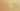MapToBooleanWithTernaryOperator

Misconception:

The ternary conditional operator is necessary for converting an expression such as a > b into a bool.Incorrect

To map a boolean expression to a bool, a ternary conditional operator is necessary

Correct

To map a boolean expression to a bool, one can just use it

CorrectionHere is what's right.

In many cases, the condition used in a ternary conditional operator is a boolean expression. In such cases, that expression already will evaluate to a bool value. Taking that bool value and using a ternany operator to map from it to a bool value is entirely unnecessary.

For example, if CONDITION is a boolean expression, then this:

b = True if CONDITION else False

can be refactored into this:

b = CONDITION

Note that Python allows non-booleans to be used as conditions in ternary conditional operators. For example, the following is an idiomatic way to check whether a list, lst in this snippet, is empty or not:

non_empty = True if lst else False

and is not equivalent to

non_empty = lst

OriginWhere could this misconception come from?

This misconception may stem from the student doing a case analysis:

• If this condition is True, what do we want to do?
• If the condition is False, what do we want to do?

SymptomsHow do you know your students might have this misconception?

In the following examples, assume that CONDITION is a boolean expression (e.g, is_happy(), i < len(lst), o is None, pacman.is_hungry(), or a and b for boolean a and b)

Example: Assignment

b = True if CONDITION else False

Example: Return

def predicate():
...
return True if CONDITION else False

Python